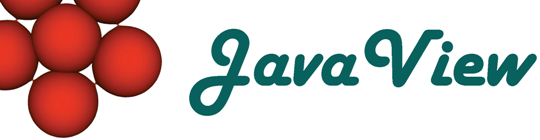Overview  Animation  Classic Surfaces  Parm Surfaces  Curves on Surfaces  Discrete Geodesics  ODE  Platonic Solids  Cycloid  Root Finder  Harmonic Maps  Rivara Bisection  Scalar Field  Weierstrass  Closed Polygon  Elastic Curve  Billiard in an Ellipse  LIC Visualization  Discrete VF  Hodge Splitting  Textured Surface  Surfaces of Rotation  Mean Curvature Flow  Pythagoraen Tree  Julia Sets

## Discrete Tangential Vector Fields

This applet handles a problem that occurs if you define a tangential discrete vector field at the vertices of a triangulation. Tangential means that every vector lies in one of the planes that are defined by the adjacent elements. So every vector is in general only tangential to one element. We want to find the best representative tangential vectors on the other elements of one vertex star.

This applet compares four different methods that try to solve this problem (more or less successfully). It creates a (mostly) random vector field on the model that is imported by the user by the 'Models' panel and then applies one of the methods to generate integral lines to this vector field.

 projection The vector at a vertex is treated to be a 3-dimensial vector and is then projected onto the plane of the element to get the representative vector belonging to this element. Creates interesting artifacts especially at vertices with high curvature. geodesic completion Generates the representative vector of an element by rotating the original vector in the vertex star to the element  and then rotating back the same angle in the elements plane. geodesic translation (half angle) Determines the representative vector of an element by geodesic translation of the original vector along the bisector of the element. That is some sort of discrete version of the continuous geodesic translation method below. geodesic translation (continuous) Determines the representative vector of an element by geodesic translation of the original vector along the connection between the vertex and the point on the element in which the vector field shall be evaluated. So each element has not only one representative vector but a continuous representative vector field.

You can look at an integral line of the current vector field by pressing 'i' and picking an initial point on the surface the same time. Parameters 'Length' and 'Discr' control this integral line.

Create a LIC texture image of the vector field by pressing the button 'calculate LIC texture'.